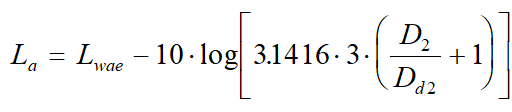Hydrodynamic Noise: IEC Standard - Intergraph Smart Instrumentation - 13.1 - Reference - Hexagon PPM

Integraph Smart Instrumentation Sizing Equations

Language
English (United States)
Product
Intergraph Smart Instrumentation
Search by Category
Reference
Smart Instrumentation Version
13.1

*D2 [m], P1 [Pa-a], P2 [Pa-a], PV [Pa-a], Pc [Pa-a], W[kg/s]

1. Calculate the downstream speed of sound: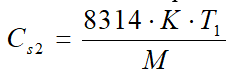2. Calculate characteristic pressure ratio: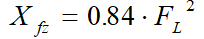3. Calculate Differential pressure ratio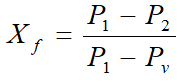4. Calculate Ring frequency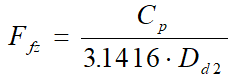5. Calculate Internal sound power level

• Case Xr<Xfz - Non-cavitating flow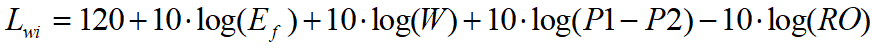• Case Xf=> Xfz - Cavitating flow

1. Calculate Liquid critical pressure ratio factor

1.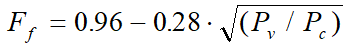2. Calculate Differential pressure

1.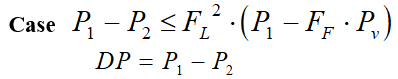2.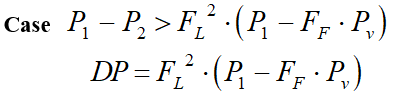3. Calculate Lwi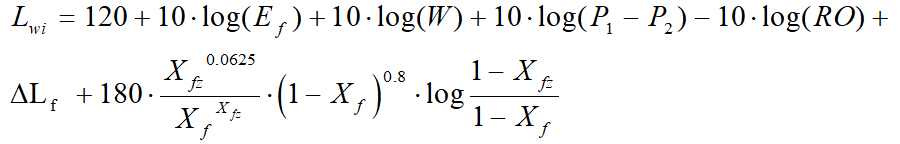6. Calculate Un-weighted extenral sound power levels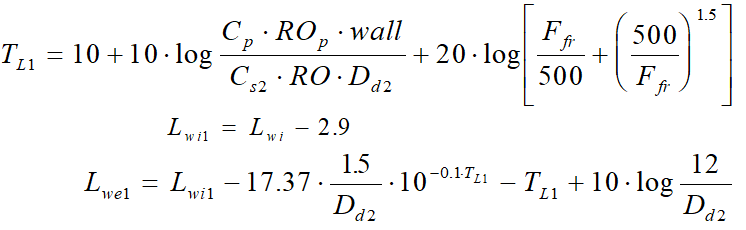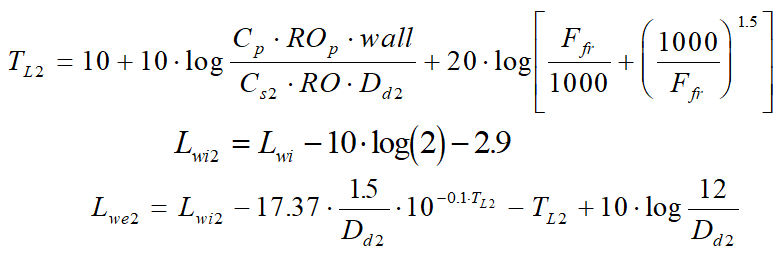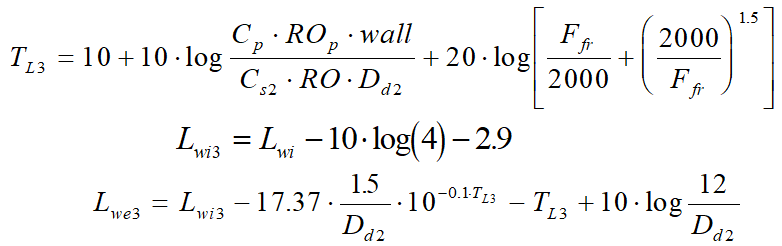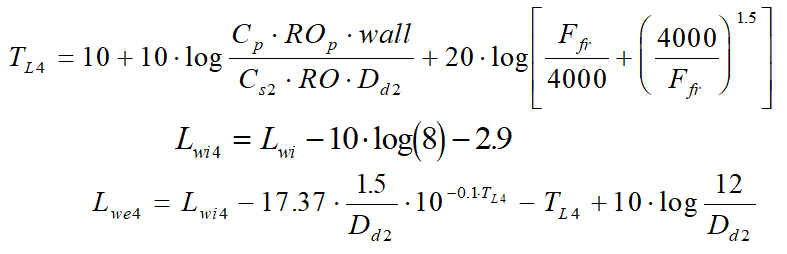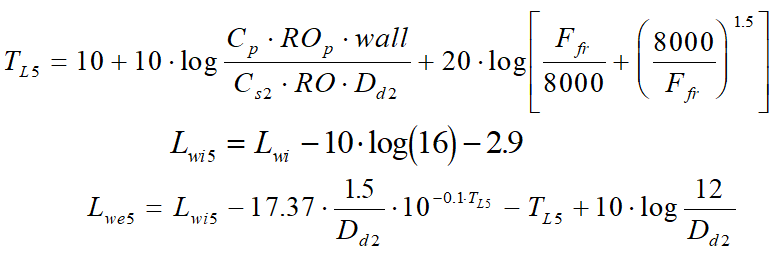7. Calculate External A-weighted sound power level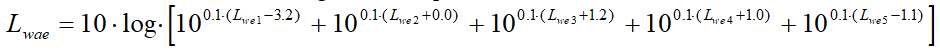8. Calculate External A-weighted sound pressure level